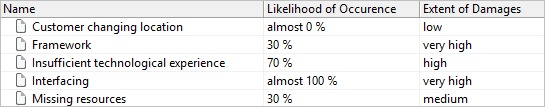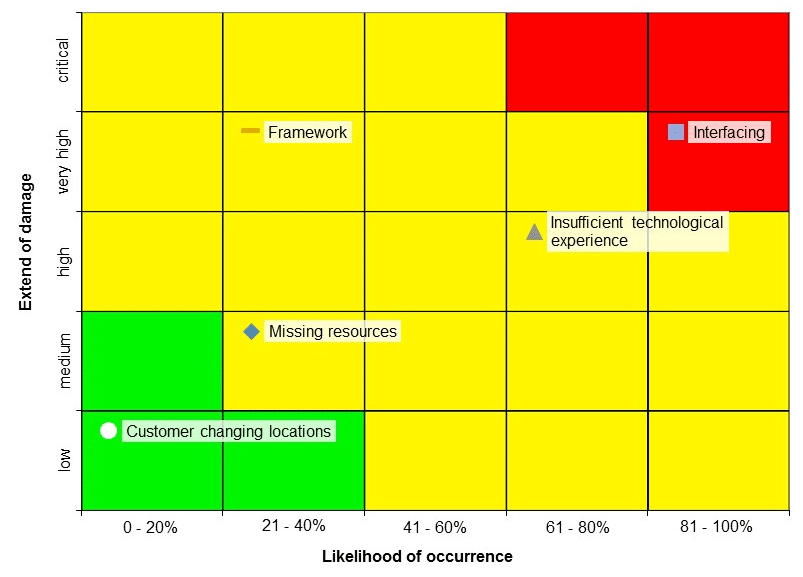# What Is a Risk Matrix?

## Risk Matrix. Visualize Risks in a Diagram.

What is a risk matrix? How is it created and what advantages does it offer?

## What Is a Risk Matrix?

A risk matrix (also called a risk diagram) visualizes risks in a diagram. In the diagram, the risks are divided depending on their likelihood and their effects or the extent of damage, so that the worst case scenario can be determined at a glance.

In this sense, the risk matrix should be seen as a result of the risk analysis and risk evaluation and is therefore an important component of your project and risk management.

## Advantages of the Risk Matrix

The risk matrix:

• Identifies the gravest project risks.
• Creates and presents the risk situation with minimal effort (e.g. as an Excel diagram).
• Presents the risk situation visually and comprehensively.
• Presents the risk situation simply for everyone because no prior knowledge is required to understand it.
• Assesses the efficiency of your risk measures.

A short definition of a risk matrix:

## How Do You Create a Risk Matrix?

To create a risk matrix or a risk diagram, the probability of occurrence and the extent of the damage have to be evaluated. Then the individual risks are entered into a coordinate system according to these values.

Evaluation of the likelihood of occurrence

There are five levels of entering the likelihood of occurrence. These levels can be expressed in percentages or in semantic concepts. For example:

0-20%, 21-40%, 41-60%, 61-80% and 81-100%
impossible, unlikely, possible, likely and highly likely

The criteria for the level of likelihood where a risk is situated has to be defined precisely. If you have quantative data, then you can base it on that. Even the reference value should be clearly defined. For example, take the expected time until the onset of the damage or the likelihood per customer. An “impossible” likelihood level is recommended so as to not have to identify the same risks again during a project, for example, if the process changes.

Evaluation of the extent of damages

In the same way, the extent of damages can be formulated in five levels, for example, low, middle, high, very high and critical.

Of course, here each level of a damage extent has to be described exactly in order to allocate the corresponding risks. For example you have to take into account an event happening that could lead to undesired results or have long or short term consequences.

The reference value is then established (for example, Euros per occurence.)

## Presentation and Example of a Risk Matrix

Exactly how the risk diagram looks is not standardized. Through the five level evaluation, a diagram often results that consists of 25 fields. The labelling of the x and y axes of the risk matrix are not determined. So you determine the y-axis as either the likelihood of occurrence or the extent of damage.

It is always important to have different colours – normally green, yellow and red – to distinguish the risks according to the likelihood that they will happen and the extent of the damage they would cause.

Because the risk diagram is only suitable for a visualization of a limited number of risks – otherwise the overview will be lost – you can also just visualize selected risks, like the top ten. Here in the example, the following risks are sorted  according to the likelihood that they will occur and the damage that they might cause:Then a very simply kept risk matrix/diagram could look like this:## Knowledge to go

Identifying and mitigating risks with a tool

• Whitepaper
• Tips & Tricks
• Software

## The Risk Matrix Has Been Created – Do You Have to Derive Measures for All Risks?

If you consider the previous example, then there are exactly three colours and each risk can be placed in a tile with a unique color. You will come across this presentation more often for a risk matrix. What exactly do the colors mean?

Green: Areas where no further measures for risk reduction are necessary
Yellow: Areas where the risks have been reduced as much as possible (also known as the “ALARP area”: As Low As Reasonably Practicable)
Red: Areas where risks are not acceptable in any case

This division is not always sensible, but it makes the process of risk management more transparent. If you find risks in the red area, then you have to take recommended measures to bring it into the ALARP area. If that is not possible, then carry out a cost-benefit analysis for the revelant risks.

Normally you cannot reduce the extent of damage of a risk through your measures – but the likelihood of occurence. So, if you determine an evaluation level for the likelihood of your risks occurring and use that to determine a risk diagram, you should pay attention to the following:

If a risk has the lowest likelihood of occurring, but the highest extent of damage, it should land at least in the yellow section of the risk matrix. So it is still acceptable there.

Create a risk matrix for your projects

Risk management is a mandatory requirement for the successful completion of a project. If risks are neither recognized nor sufficiently evaluated, you can’t take any recommended measures. It is not only important for you to be as informed as possible on the risks, but also the other project participants, like stakeholders. How can you create an overview of the risks? Simply, by using a tool with which you can record risks, evaluate the likelihood that they will occur and the extent of the damage, and generate the information as a risk matrix in an Excel document.